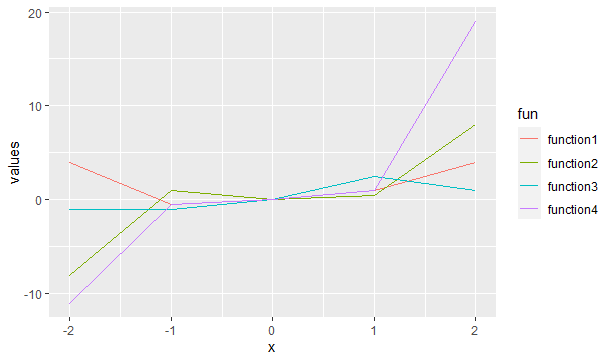Open In App

# Create Legend in ggplot2 Plot in R

In this article, we will discuss how to create a legend in ggplot using R programming language. To draw a legend within ggplot the parameter col is used, it basically adds colors to the plot and these colors are used to differentiate between different plots. To depict what each color represents a legend is produced by ggplot. col attribute can be specified in 2 places.

### Method 1: Specifying col in ggplot()

Simply specifying on basis of which attribute colors should be differentiated to the col attribute within ggplot() will get the job done.

Syntax: ggplot(df, aes(x, y, col=”name of the column to differentiate on the basis of”))

Code:

## R

 `library``(``"ggplot2"``)`` ` ` ` `function1 <- ``function``(x){x**2}``function2 <- ``function``(x){x**3}``function3 <- ``function``(x){x/2}``function4 <- ``function``(x){2*(x**3)+(x**2)-(x/2)}`` ` `df=``data.frame``(x = -2:2,``              ``values=``c``(``function1``(-2 : 2),``                       ``function2``(-2 : 2),``                       ``function3``(-2 : 2),``                       ``function4``(-2 : 2)),``              ``fun=``rep``(``c``(``"function1"``, ``"function2"``,``                        ``"function3"``,``"function4"``))``)`` ` `ggplot``(df,``aes``(x,values,col=fun))+``geom_line``()`

Output:### Method 2: Using col in geom

The same can be done inside any geom function. In the example given below, it has been applied to geom_line() but the same can be done with any other geom function as per requirement.

Syntax: geom_function(aes(col=”name of the column to differentiate upon”))

Code:

## R

 `library``(``"ggplot2"``)`` ` ` ` `function1 <- ``function``(x){x**2}``function2 <- ``function``(x){x**3}``function3 <- ``function``(x){x/2}``function4 <- ``function``(x){2*(x**3)+(x**2)-(x/2)}`` ` `df=``data.frame``(x = -2:2,``              ``values = ``c``(``function1``(-2:2),``                       ``function2``(-2:2),``                       ``function3``(-2:2),``                       ``function4``(-2:2)),``              ``fun=``rep``(``c``(``"function1"``,``"function2"``,``                        ``"function3"``,``"function4"``))``)`` ` `ggplot``(df, ``aes``(x, values)) + ``geom_line``(``aes``(col = fun))`

Output: